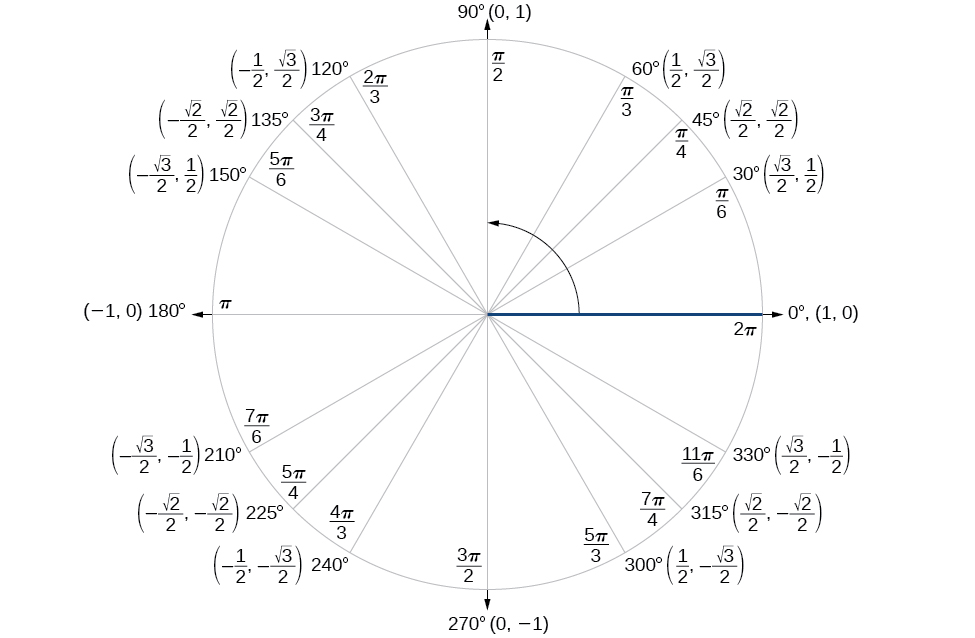# 9.2 Sum and difference identities

 Page 1 / 6
In this section, you will:
• Use sum and difference formulas for cosine.
• Use sum and difference formulas for sine.
• Use sum and difference formulas for tangent.
• Use sum and difference formulas for cofunctions.
• Use sum and difference formulas to verify identities.Mount McKinley, in Denali National Park, Alaska, rises 20,237 feet (6,168 m) above sea level. It is the highest peak in North America. (credit: Daniel A. Leifheit, Flickr)

How can the height of a mountain be measured? What about the distance from Earth to the sun? Like many seemingly impossible problems, we rely on mathematical formulas to find the answers. The trigonometric identities, commonly used in mathematical proofs, have had real-world applications for centuries, including their use in calculating long distances.

The trigonometric identities we will examine in this section can be traced to a Persian astronomer who lived around 950 AD, but the ancient Greeks discovered these same formulas much earlier and stated them in terms of chords. These are special equations or postulates, true for all values input to the equations, and with innumerable applications.

In this section, we will learn techniques that will enable us to solve problems such as the ones presented above. The formulas that follow will simplify many trigonometric expressions and equations. Keep in mind that, throughout this section, the term formula is used synonymously with the word identity .

## Using the sum and difference formulas for cosine

Finding the exact value of the sine, cosine, or tangent of an angle is often easier if we can rewrite the given angle in terms of two angles that have known trigonometric values. We can use the special angles , which we can review in the unit circle shown in [link] .The Unit Circle

We will begin with the sum and difference formulas for cosine , so that we can find the cosine of a given angle if we can break it up into the sum or difference of two of the special angles. See [link] .

 Sum formula for cosine $\mathrm{cos}\left(\alpha +\beta \right)=\mathrm{cos}\text{\hspace{0.17em}}\alpha \text{\hspace{0.17em}}\mathrm{cos}\text{\hspace{0.17em}}\beta -\mathrm{sin}\text{\hspace{0.17em}}\alpha \text{\hspace{0.17em}}\mathrm{sin}\text{\hspace{0.17em}}\beta$ Difference formula for cosine $\mathrm{cos}\left(\alpha -\beta \right)=\mathrm{cos}\text{\hspace{0.17em}}\alpha \text{\hspace{0.17em}}\mathrm{cos}\text{\hspace{0.17em}}\beta +\mathrm{sin}\text{\hspace{0.17em}}\alpha \text{\hspace{0.17em}}\mathrm{sin}\text{\hspace{0.17em}}\beta$

First, we will prove the difference formula for cosines. Let’s consider two points on the unit circle. See [link] . Point $\text{\hspace{0.17em}}P\text{\hspace{0.17em}}$ is at an angle $\text{\hspace{0.17em}}\alpha \text{\hspace{0.17em}}$ from the positive x- axis with coordinates $\text{\hspace{0.17em}}\left(\mathrm{cos}\text{\hspace{0.17em}}\alpha ,\mathrm{sin}\text{\hspace{0.17em}}\alpha \right)\text{\hspace{0.17em}}$ and point $\text{\hspace{0.17em}}Q\text{\hspace{0.17em}}$ is at an angle of $\text{\hspace{0.17em}}\beta \text{\hspace{0.17em}}$ from the positive x- axis with coordinates $\text{\hspace{0.17em}}\left(\mathrm{cos}\text{\hspace{0.17em}}\beta ,\mathrm{sin}\text{\hspace{0.17em}}\beta \right).\text{\hspace{0.17em}}$ Note the measure of angle $\text{\hspace{0.17em}}POQ\text{\hspace{0.17em}}$ is $\text{\hspace{0.17em}}\alpha -\beta .\text{\hspace{0.17em}}$

Label two more points: $\text{\hspace{0.17em}}A\text{\hspace{0.17em}}$ at an angle of $\text{\hspace{0.17em}}\left(\alpha -\beta \right)\text{\hspace{0.17em}}$ from the positive x- axis with coordinates $\text{\hspace{0.17em}}\left(\mathrm{cos}\left(\alpha -\beta \right),\mathrm{sin}\left(\alpha -\beta \right)\right);\text{\hspace{0.17em}}$ and point $\text{\hspace{0.17em}}B\text{\hspace{0.17em}}$ with coordinates $\text{\hspace{0.17em}}\left(1,0\right).\text{\hspace{0.17em}}$ Triangle $\text{\hspace{0.17em}}POQ\text{\hspace{0.17em}}$ is a rotation of triangle $\text{\hspace{0.17em}}AOB\text{\hspace{0.17em}}$ and thus the distance from $\text{\hspace{0.17em}}P\text{\hspace{0.17em}}$ to $\text{\hspace{0.17em}}Q\text{\hspace{0.17em}}$ is the same as the distance from $\text{\hspace{0.17em}}A\text{\hspace{0.17em}}$ to $\text{\hspace{0.17em}}B.$

We can find the distance from $\text{\hspace{0.17em}}P\text{\hspace{0.17em}}$ to $\text{\hspace{0.17em}}Q\text{\hspace{0.17em}}$ using the distance formula    .

$\begin{array}{ccc}\hfill {d}_{PQ}& =& \sqrt{{\left(\mathrm{cos}\text{\hspace{0.17em}}\alpha -\mathrm{cos}\text{\hspace{0.17em}}\beta \right)}^{2}+{\left(\mathrm{sin}\text{\hspace{0.17em}}\alpha -\mathrm{sin}\text{\hspace{0.17em}}\beta \right)}^{2}}\hfill \\ & =& \sqrt{{\mathrm{cos}}^{2}\alpha -2\text{\hspace{0.17em}}\mathrm{cos}\text{\hspace{0.17em}}\alpha \text{\hspace{0.17em}}\mathrm{cos}\text{\hspace{0.17em}}\beta +{\mathrm{cos}}^{2}\beta +{\mathrm{sin}}^{2}\alpha -2\text{\hspace{0.17em}}\mathrm{sin}\text{\hspace{0.17em}}\alpha \text{\hspace{0.17em}}\mathrm{sin}\text{\hspace{0.17em}}\beta +{\mathrm{sin}}^{2}\beta }\hfill \end{array}$

Then we apply the Pythagorean identity and simplify.

$\begin{array}{cc}=& \sqrt{\left({\mathrm{cos}}^{2}\alpha +{\mathrm{sin}}^{2}\alpha \right)+\left({\mathrm{cos}}^{2}\beta +{\mathrm{sin}}^{2}\beta \right)-2\text{\hspace{0.17em}}\mathrm{cos}\text{\hspace{0.17em}}\alpha \text{\hspace{0.17em}}\mathrm{cos}\text{\hspace{0.17em}}\beta -2\text{\hspace{0.17em}}\mathrm{sin}\text{\hspace{0.17em}}\alpha \text{\hspace{0.17em}}\mathrm{sin}\text{\hspace{0.17em}}\beta }\hfill \\ =& \sqrt{1+1-2\text{\hspace{0.17em}}\mathrm{cos}\text{\hspace{0.17em}}\alpha \text{\hspace{0.17em}}\mathrm{cos}\text{\hspace{0.17em}}\beta -2\text{\hspace{0.17em}}\mathrm{sin}\text{\hspace{0.17em}}\alpha \text{\hspace{0.17em}}\mathrm{sin}\text{\hspace{0.17em}}\beta }\hfill \\ =& \sqrt{2-2\text{\hspace{0.17em}}\mathrm{cos}\text{\hspace{0.17em}}\alpha \text{\hspace{0.17em}}\mathrm{cos}\text{\hspace{0.17em}}\beta -2\text{\hspace{0.17em}}\mathrm{sin}\text{\hspace{0.17em}}\alpha \text{\hspace{0.17em}}\mathrm{sin}\text{\hspace{0.17em}}\beta }\hfill \end{array}$

Similarly, using the distance formula we can find the distance from $\text{\hspace{0.17em}}A\text{\hspace{0.17em}}$ to $\text{\hspace{0.17em}}B.$

#### Questions & Answers

A laser rangefinder is locked on a comet approaching Earth. The distance g(x), in kilometers, of the comet after x days, for x in the interval 0 to 30 days, is given by g(x)=250,000csc(π30x). Graph g(x) on the interval [0, 35]. Evaluate g(5)  and interpret the information. What is the minimum distance between the comet and Earth? When does this occur? To which constant in the equation does this correspond? Find and discuss the meaning of any vertical asymptotes.
Kaitlyn Reply
The sequence is {1,-1,1-1.....} has
amit Reply
circular region of radious
Kainat Reply
how can we solve this problem
Joel Reply
Sin(A+B) = sinBcosA+cosBsinA
Eseka Reply
Prove it
Eseka
Please prove it
Eseka
hi
Joel
June needs 45 gallons of punch. 2 different coolers. Bigger cooler is 5 times as large as smaller cooler. How many gallons in each cooler?
Arleathia Reply
7.5 and 37.5
Nando
find the sum of 28th term of the AP 3+10+17+---------
Prince Reply
I think you should say "28 terms" instead of "28th term"
Vedant
the 28th term is 175
Nando
192
Kenneth
if sequence sn is a such that sn>0 for all n and lim sn=0than prove that lim (s1 s2............ sn) ke hole power n =n
SANDESH Reply
write down the polynomial function with root 1/3,2,-3 with solution
Gift Reply
if A and B are subspaces of V prove that (A+B)/B=A/(A-B)
Pream Reply
write down the value of each of the following in surd form a)cos(-65°) b)sin(-180°)c)tan(225°)d)tan(135°)
Oroke Reply
Prove that (sinA/1-cosA - 1-cosA/sinA) (cosA/1-sinA - 1-sinA/cosA) = 4
kiruba Reply
what is the answer to dividing negative index
Morosi Reply
In a triangle ABC prove that. (b+c)cosA+(c+a)cosB+(a+b)cisC=a+b+c.
Shivam Reply
give me the waec 2019 questions
Aaron Reply

### Read also:

#### Get the best Algebra and trigonometry course in your pocket!

Source:  OpenStax, Algebra and trigonometry. OpenStax CNX. Nov 14, 2016 Download for free at https://legacy.cnx.org/content/col11758/1.6
Google Play and the Google Play logo are trademarks of Google Inc.

Notification Switch

Would you like to follow the 'Algebra and trigonometry' conversation and receive update notifications?ByBy Mariah HauptmanBy